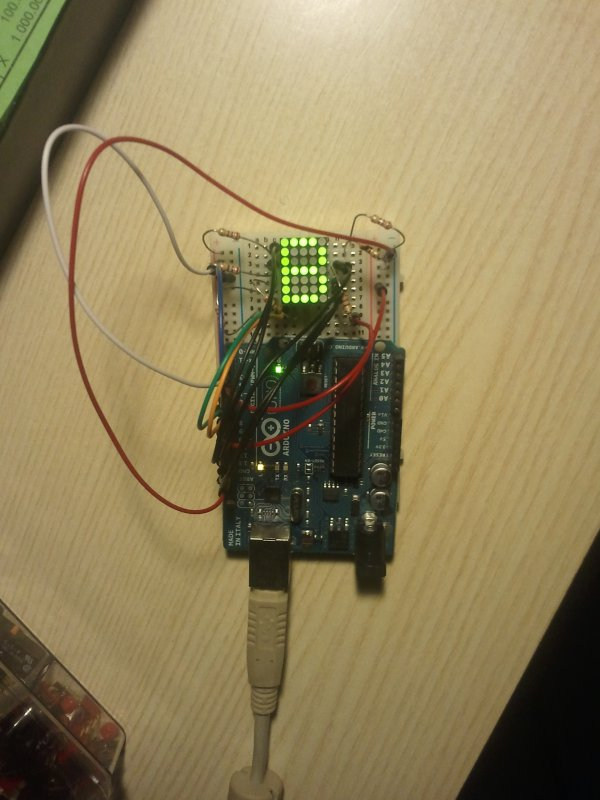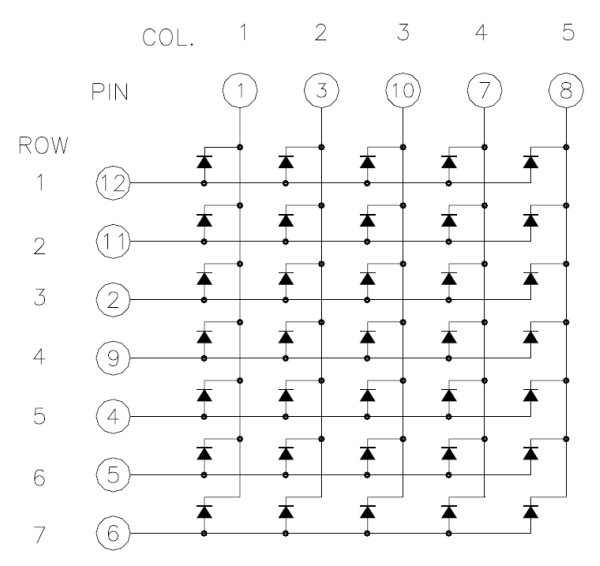# Drawing on a 7×5 LED matrix with Arduino in C

In my component drawers I have a LTP-7357AG, which is a matrix of 35 green LEDs conveniently packaged in a 12-pin display. I wanted to play with it so I began to hook it with my Arduino Uno. This post is part of a series about programming Arduino applications in C.

I’m going to show the results first, and then give an explanation of what I did and why. The following image shows that the Arduino is capable of showing a letter on the display.

I made this by writing a library in C that handles the LED matrix when it’s connected to particular I/O ports, and together with the library I wrote a main program that displays the “B”:`#include &lt;util/delay.h&gt;`
`#include &quot;ledmatrix.h&quot; // The header of my library.`
`#define FRAME_RATE_HZ 50 // This feels sufficient`
`#define SUBFRAME_RATE_HZ (FRAME_RATE_HZ * N_SUBFRAMES) // N_SUBFRAMES is in ledmatrix.h`
`#define SUBFRAME_DELAY_US (1000000 / SUBFRAME_RATE_HZ)`
`int` `main(``void``)`
`{`
` ``struct` `ledmatrix_frame fB = ``// struct ledmatrix is a 5-bytes struct holding a 7x5 frame.`
`  ``LEDMATRIX_FRAME_INIT( ``// A helper macro to write frames easily`
`   ``11110,  ``// This structure contains a &quot;B&quot;`
`   ``10001,  ``// 1 means LED is on`
`   ``10001,  ``// 0 means LED is off`
`   ``11110,  ``// they are transformed into binary numbers by &quot;magic&quot; in LEDMATRIX_FRAME_INIT()`
`   ``10001,`
`   ``10001,`
`   ``11110);`
` ``ledmatrix_setup(); ``// Initializes ports and stuff.`
` ``while``(1)`
` ``{`
`  ``ledmatrix_draw_next_subframe(&amp;fB); ``// Turns on the LEDs of the next subframe.`
`  ``_delay_us(SUBFRAME_DELAY_US); ``// This loop+delay might be replaced by a periodic interrupt.`
` ``}`
` ``return` `0;`
`}`

This is the internal diagram of the component:

The elemental principle for each dot of the display is that if I drive row 1 high and drive column 1 low, I will light up the dot at coordinates (1,1), and if I drive row 1 low and column 1 low I will keep it off.

In the tutorials that can be found online on how to drive a LED matrix, they usually scan the matrix by row or by column. I decided to scan by column, so I hooked one resistor for each row pin. The LED matrix datasheet indicates that each LED has a forward voltage of 2.1V at the typical operating current of 20mA. Given the 5V output of the Arduino I/O, this suggests to use a resistor of around 145Ohm, but I used 220Ohm resistors because that’s what I had available; the downside is that the LED will be less bright because less current will pass through it, nothing dramatic though.

I wanted to connect the LED matrix pins as orderly as possible in terms of Arduino pin numbers, so I started from pin 0 that corresponds to `PORTD0` of the ATmega chip, but I had problems with pins 0 and 1 probably caused by the connection to the USB-serial converter mounted on the Arduino Uno.

This is the final wiring of the Arduino to the component:

Note that the colours of the wires match the photo at the beginning of this post. This is a table that explicitly indicates the connection:

```LED matrix  - Arduino
COL1(PIN1)  - PB0(PIN8)
COL2(PIN3)  - PB1(PIN9)
COL3(PIN10) - PB2(PIN10)
COL4(PIN7)  - PB3(PIN11)
COL5(PIN8)  - PB4(PIN12)
ROW1(PIN12) - PB5(PIN13)
ROW2(PIN11) - PD7(PIN7)
ROW3(PIN2)  - PD2(PIN2)
ROW4(PIN9)  - PD3(PIN3)
ROW5(PIN4)  - PD4(PIN4)
ROW6(PIN5)  - PD5(PIN5)
ROW7(PIN6)  - PD6(PIN6)```

In many examples, when performing a column scan, the active column is driven low by a pin, while the others are not driven: the I/O of the Arduino are configured as high-Z input. In this way one can light up each dot of the column by driving high or low the corresponding pins. The problem with driving 7 dots at once is that the Arduino I/O that keeps the column pin low is sinking too much current: the ATmega datasheet indicates a maximum current of 40mA per pin, but each dot needs roughly 20mA, so we should at most light 2 dots. Some people suggest using a current sink IC to solve this problem, but I don’t have such an IC yet in my inventory, so I decided to consider 2 dots at a time instead of a full column; the dots considered at one time is what is called “subframe” in the code. The following GIF shows the scan of a “B” with a column scan that considers at most 2 dots at a time:

READ  Best RGB LEDs for any project (WS2812)

For more detail: Drawing on a 7×5 LED matrix with Arduino in C

#### This Post / Project can also be found using search terms:

• 5 by 7 matrix arduino
• 7*5 ledmatrix
• ledmatrix arduino 8×16